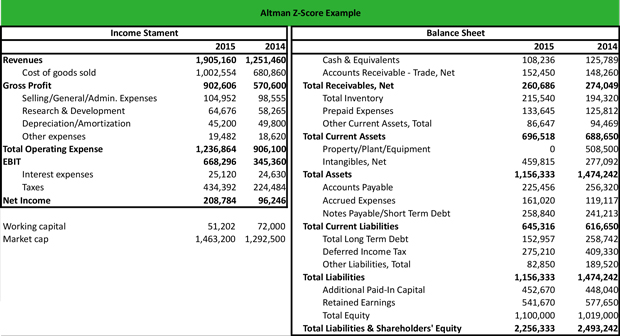# What is the Altman Z-Score?

Definition: The Altman Z-score is a financial formula used by investors and creditors to to estimate the likelihood of the company going bankrupt by taking into account a firm’s core activities, liquidity, solvency, profitability and leverage.

## What Does Altman Z Score Mean?

What is the definition of Altman Z score? By evaluating a firm’s working capitaltotal assets, total liabilitiesretained earnings, operating earnings, and revenues, the Altman Z-score is a reliable predictor of a firm’s solvency. Analysts often equate the this score with corresponding bond ratings because if a firm has a bond rating of BBB, it will have a score that will signify bankruptcy.

Also, Z score is one of the most accurate credit models because a change in a firm’s score suggests that, most likely, the firm’s fundamentals have changed. The Altman Z-score formula is calculated like this:

1.2A + 1.4B + 3.3C + 0.6D + 1.0E

Here are each of the components broken down.

A = WC / TA = Working Capital / Total Assets

B = RE / TA = Retained Earnings / Total Assets

C = EBIT / TA = EBIT / Total Assets

D = MC / TL = Market Cap / Total Liabilities

E = S / TA = Sales / Total Assets

Let’s look at an example.

## Example

Michael is a financial analyst at a boutique securities firm. He is asked to calculate the score for a manufacturing company that has released relatively weak results over the past two quarters. Michael collects information from the following balance sheet and income statement:Z-Score = ((WC/TA) x 1.2) + ((RE/TA) x 1.4) + ((EBIT/TA) x 3.3) + ((MC/TL) x 0.6) + ((S/TA) x 1.0) = 0.053 + 0.656 + 1.907 + 0.759 + 1.648 = 5.023.

Since the firm has a Z-score higher than 3.0, it is financially strong, and the likelihood of bankruptcy is low. The company has a positive working capital, suggesting that it can meet its short-term obligations with its current assets. Its retained earnings indicate that the company has a relatively low leverage, which allows it to finance its core operations with equity.

Furthermore, the company has strong EBIT, suggesting strong efficiency, and high revenues, suggesting an efficient use of its assets.

## Summary Definition

Define Altman Z-Score: The Altman score is a financial ratio that calculates the liquidity of a company and helps analysts predict the likelihood that the firm will go bankrupt.